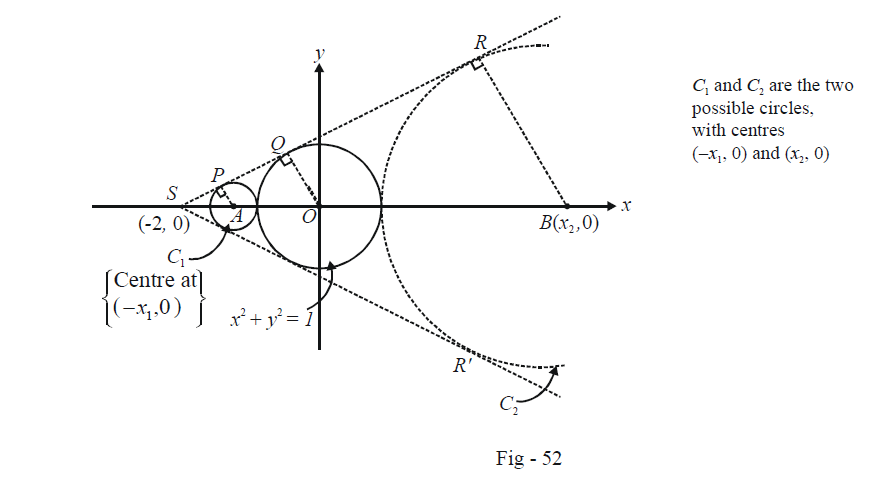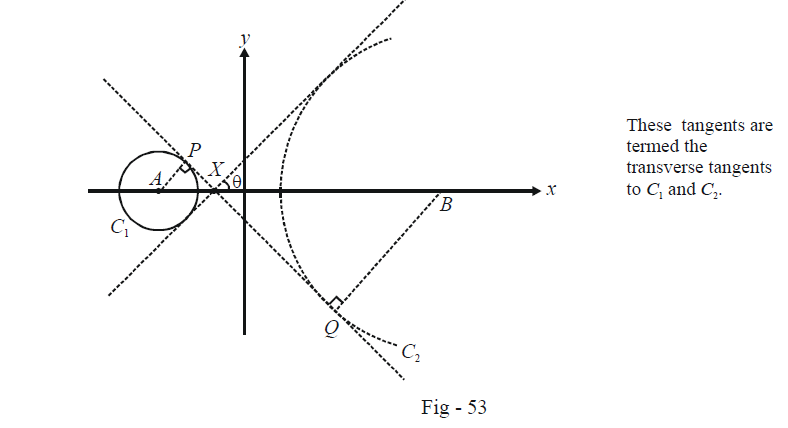# Circles Set-2

Go back to  'SOLVED EXAMPLES'

Example – 4

Let $${T_1}$$ and $${T_2}$$ be two tangents drawn from (–2, 0) to the circle $${x^2} + {y^2} = 1.$$ Determine the circles touching C and having $${T_1}$$  and $${T_2}$$  as their pair of tangents. Also find all the possible pair wise common tangents to these circles.

Solution: Note that two such circles can be drawn, as shown in the figure below:Let the radii of $${C_1}$$ and $${C_2}$$ be $${r_1}$$ and $${r_2}$$ respectively. We can evaluate the coordinates of both A and B very easily from geometrical considerations. We have

\begin{align}&\qquad\quad \Delta ASP \sim \Delta OSQ \sim \Delta BSR\\& \Rightarrow \quad \frac{{AS}}{{AP}} = \frac{{OS}}{{OQ}} = \frac{{BS}}{{BR}}\end{align}

Now,                                                                                          $$AS = 2 - {x_1},\,\,AP = {r_1},\,\,OS = 2,\,\,OQ = 1,\,\,BS = 2 + {x_2},\,\,BR = {r_2}$$

$\Rightarrow \quad \frac{{2 - {x_1}}}{{{r_1}}} = \frac{2}{1} = \frac{{2 + {x_2}}}{{{r_2}}}$

Also, note that $${x_1} = 1 + {r_1}$$ and $${x_2} = 1 + {r_2}.$$ Thus,

\begin{align}\Rightarrow \quad \frac{{2 - {x_1}}}{{{x_1} - 1}} = 2 = \frac{{2 + {x_2}}}{{{x_2} - 1}}\end{align}

\begin{align} \Rightarrow \quad {x_1} = \frac{4}{3} \;and \;{x_2} = 4 \end{align}

\begin{align}\Rightarrow \quad {r_1} = \frac{1}{3} \; and \; {r_2} = 3 \end{align}

Thus, the equations to $${C_1}$$ and $${C_2}$$ are

\begin{align}{C_1}:{\left( {x + \frac{4}{3}} \right)^2} + {y^2} = {\left( {\frac{1}{3}} \right)^2}\\{C_2}:{(x - 4)^2} + {y^2} = {3^2}\end{align}

Now we determine all the possible common tangents to $${C_1}$$ and $${C_2}$$ .

Two such possible tangents are simply $$SR$$ and $$SR'$$ which both touch $${C_1}$$ and $${C_2}$$ on the same side. There will be two other possible common tangents, each having A and B on the opposite side of it as shown in the figure below:Let X have the co-ordinates $$(x,0).$$ Note that $$\Delta APX \sim \Delta BQX.$$

Thus,

\begin{align}&\,\,\,\,\,\,\,\,\,\,\,\,\,\frac{{AX}}{{AP}} = \frac{{BX}}{{BQ}}\\&\Rightarrow \quad \frac{{x + \frac{4}{3}}}{{1/3}} = \frac{{4 - x}}{3}\\&\Rightarrow \quad x = - \frac{4}{5}\end{align}

Also,

\begin{align}& \qquad \quad\;\; \sin \theta =\frac{AP}{AX}=\frac{1/3}{-4/5+4/3}=\frac{5}{8} \\ & \Rightarrow \qquad \tan \theta =\frac{5}{\sqrt{{{8}^{2}}-{{5}^{2}}}}=\frac{5}{\sqrt{39}} \\ \end{align}

Thus, the two transverse tangents pass through \begin{align}\left( {\frac{{ - 4}}{5},0} \right)\end{align} and have slopes \begin{align} \pm \frac{5}{{\sqrt {39} }}.\end{align} Their equations are therefore

$y = \pm \frac{5}{{\sqrt {39} }}\left( {x + \frac{4}{5}} \right)$

Example – 5

Find the values of a for which the line $$x + y = 0$$ bisects two chords drawn from a point \begin{align}P\left( {\frac{{1 + \sqrt 2 a}}{2},\frac{{1 - \sqrt 2 a}}{2}} \right)\end{align} to the circle \begin{align}S:{x^2} + {y^2} - \left( {\frac{{1 + \sqrt 2 a}}{2}} \right)x - \left( {\frac{{1 - \sqrt 2 a}}{2}} \right)y = 0\end{align}.

Solution: This question is somewhat similar to Example -3. Note that the point P lies on S.

Since the mid-point M of the chord(s) drawn from P lies on $$x + y = 0,$$ we can assume its co-ordinates to be $$(h, - h).$$ The equation of the chord of S bisected at M then becomes:

\begin{align}&\qquad\;\; T(h, - h) = S(h, - h)\\&\Rightarrow \quad hx - hy - \frac{\lambda }{2}(x + h) - \frac{{\bar \lambda }}{2}(y - h) = 2{h^2} - \lambda h + \bar \lambda h\,\,\,\,\,\,\,\,\,\,\,\,\,\,\,\,\,\,\,\,\,\,\,\,\,...(1)\end{align}

where the substitution \begin{align}\lambda = \frac{{1 + \sqrt 2 a}}{2},\,\,\bar \lambda = \frac{{1 - \sqrt 2 a}}{2}\end{align}  has been done for convenience.

Since the chord (1) passes through $$P(\lambda ,\bar \lambda ),$$ its co-ordinates must satisfy (1). Thus,

\begin{align}&\qquad \quad h\lambda - h\bar \lambda - \frac{\lambda }{2}(\lambda + h) - \frac{{\bar \lambda }}{2}(\bar \lambda - h) = 2{h^2} - \lambda h + \bar \lambda h\\ &\;\Rightarrow \quad 4{h^2} - 3(\lambda - \bar \lambda )h + ({\lambda ^2} + {{\bar \lambda }^2}) = 0\,\,\,\,\,\,\,\,\,\,\,\,\,\,\,\,\,\,\,\,\,\,\,\,\,\,\,\,\,\,\,\,\,...\left( 2 \right)\end{align}

Since we want two possible chords to exist satisfying the given property, the quadratic in (2) must yield distinct values of h. Thus, its discriminant must be positive:

$9{(\lambda - \bar \lambda )^2} > 16({\lambda ^2} + {\bar \lambda ^2})$

Substituting the values of $$\lambda$$ and $$\bar \lambda$$ and simplifying, we obtain

\begin{align}&\qquad\;\; {a^2} - 4 > 0\\ & \Rightarrow \quad a \in ( - \infty , - 2) \cup (2,\infty )\end{align}

These are the required values of $$a$$.

Example –6

If $${S_1} = 0$$ and $${S_2} = 0$$ are circles having radii $${r_1}$$ and $${r_2}$$ respectively, prove that the circles \begin{align}S:\frac{{{S_1}}}{{{r_1}}} + \frac{{{S_2}}}{{{r_2}}} = 0\;\;and\;\;S':\frac{{{S_1}}}{{{r_1}}} - \frac{{{S_2}}}{{{r_2}}} = 0\end{align} intersect orthogonally.

Solution: Let the equation of the two given circles be

\begin{align}&{S_1}:{x^2} + {y^2} + 2{g_1}x + 2{f_1}y + {c_1} = 0\,\,\,;\,\,\,r_1^2 = g_1^2 + f_1^2 - {c_1}\\&{S_2}:{x^2} + {y^2} + 2{g_2}x + 2{f_2}y + {c_2} = 0\,\,\,;\,\,\,r_2^2 = g_2^2 + f_2^2 - {c_2}\end{align}

Then, the equation of S and S ' are

\begin{align}&S:({x^2} + {y^2})\left( {\frac{1}{{{r_1}}} + \frac{1}{{{r_2}}}} \right) + 2\left( {\frac{{{g_1}}}{{{r_1}}} + \frac{{{g_2}}}{{{r_2}}}} \right)x + 2\left( {\frac{{{f_1}}}{{{r_1}}} + \frac{{{f_2}}}{{{r_2}}}} \right)y + \left( {\frac{{{c_1}}}{{{r_1}}} + \frac{{{c_2}}}{{{r_2}}}} \right) = 0\\&S':({x^2} + {y^2})\left( {\frac{1}{{{r_1}}} - \frac{1}{{{r_2}}}} \right) + 2\left( {\frac{{{g_1}}}{{{r_1}}} - \frac{{{g_2}}}{{{r_2}}}} \right)x + 2\left( {\frac{{{f_1}}}{{{r_1}}} - \frac{{{f_2}}}{{{r_2}}}} \right)y + \left( {\frac{{{c_1}}}{{{r_1}}} - \frac{{{c_2}}}{{{r_2}}}} \right) = 0\end{align}

We now check the orthogonality condition for S and S', i.e. we check whether $$2({g_s}{g_{s'}} + {f_s}{f_{s'}}) = {c_s} + {c_{s'}}$$

Thus,

\begin{align}&2({g_s}{g_{s'}} + {f_s}{f_{s'}}) = 2\frac{{\left( {\frac{{{g_1}}}{{{r_1}}} + \frac{{{g_2}}}{{{r_2}}}} \right)\left( {\frac{{{g_1}}}{{{r_1}}} - \frac{{{g_2}}}{{{r_2}}}} \right)}}{{\left( {\frac{1}{{{r_1}}} + \frac{1}{{{r_2}}}} \right)\left( {\frac{1}{{{r_1}}} - \frac{1}{{{r_2}}}} \right)}} + 2\frac{{\left( {\frac{{{f_1}}}{{{r_1}}} + \frac{{{f_2}}}{{{r_2}}}} \right)\left( {\frac{{{f_1}}}{{{r_1}}} - \frac{{{f_2}}}{{{r_2}}}} \right)}}{{\left( {\frac{1}{{{r_1}}} + \frac{1}{{{r_2}}}} \right)\left( {\frac{1}{{{r_1}}} - \frac{1}{{{r_2}}}} \right)}}\\&\qquad\qquad\qquad\quad = 2\frac{{\left( {\frac{{g_1^2 + f_1^2}}{{r_1^2}} - \frac{{g_2^2 + f_2^2}}{{r_2^2}}} \right)}}{{\left( {\frac{1}{{r_1^2}} - \frac{1}{{r_2^2}}} \right)}}\,\\&\qquad\qquad\qquad\quad = 2\frac{{\left( {\frac{{{c_1}}}{{r_1^2}} - \frac{{{c_2}}}{{r_2^2}}} \right)}}{{\left( {\frac{1}{{r_1^2}} - \frac{1}{{r_2^2}}} \right)}}\qquad\qquad\qquad\qquad \qquad\qquad\qquad\quad ...(1)\end{align}

whereas

\begin{align}{c_s} + {c_{s'}} = \frac{{\frac{{{c_1}}}{{{r_1}}} + \frac{{{c_2}}}{{{r_2}}}}}{{\frac{1}{{{r_1}}} + \frac{1}{{{r_2}}}}} + \frac{{\frac{{{c_1}}}{{{r_1}}} - \frac{{{c_2}}}{{{r_2}}}}}{{\frac{1}{{{r_1}}} - \frac{1}{{{r_2}}}}}\end{align}

\begin{align} = 2\frac{{\left( {\frac{{{c_1}}}{{r_1^2}} - \frac{{{c_2}}}{{r_2^2}}} \right)}}{{\left( {\frac{1}{{r_1^2}} - \frac{1}{{r_2^2}}} \right)}}\,\,\,\,\,\,\,\,\,\,\,\,\,\,\,\,\,\,\,\,\,\,\,\,\,\,\,\,\,\,\,\,\,\,\,\,\,\,\,...(2)\end{align}

The equality of (1) and (2) implies that the two circles are orthogonal.

Learn from the best math teachers and top your exams

• Live one on one classroom and doubt clearing
• Practice worksheets in and after class for conceptual clarity
• Personalized curriculum to keep up with school